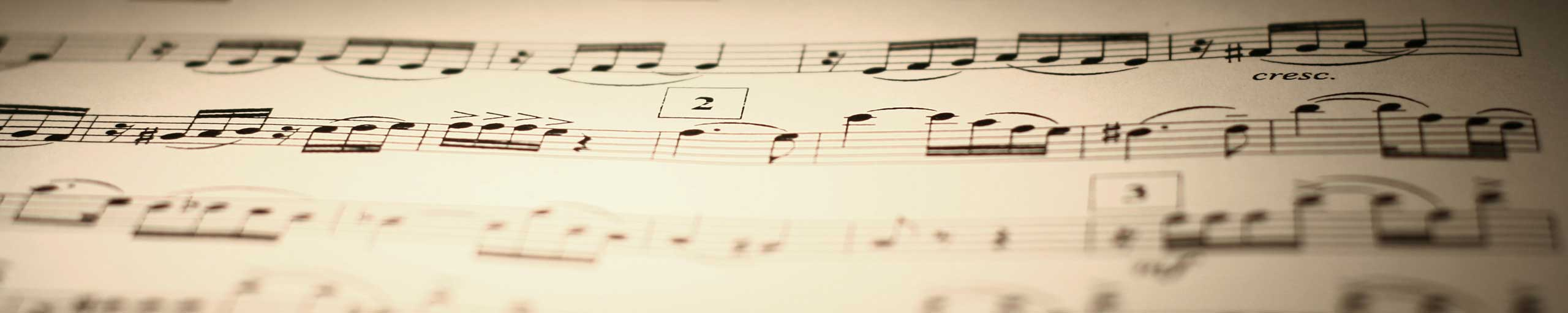# Free GRE Solutions and Explanations to Sample 1

Solutions and detailed explanations to questions and problems similar to the questions in the GRE test. sample 1

## Solution to Question 1

The number of miles covered using 1 gallon is given by
(130 / 4) = 32.5 miles/gallon
Using 6.5 gallons, the car will cover
6.7 * 32.5 = 217.75 miles

## Solution to Question 2

Shown below is the graph of ||x|-4| by steps. First top left is the V-shaped graph of |x|, then below is the graph of |x|-4 which is the graph of |x| shifted 4 units down. Top right is the graph of ||x| - 4| which is the graph of |x|-4 where the part of the graph below the x axis is reflected on the x axis to make it positive or zero. Finally the graph of ||x| - 4| and that of y = 2 shows that there are 4 points of intersection..

## Solution to Question 3

Convert all given quantities into decimal numbers
A) 125% = 125/100 = 1.25
B) 1.25 = 1.25
C) 1 + 1/3 = 1.333...
D) 4/3 = 1.333...
E) 0.0015/0.001 = 1.5/1 = 1.5
The largest of all given quantities is 0.0015/0.001

## Solution to Question 4

First calculate |- 10 - 19|
|- 10 - 19| = |-29| = 29
Substitute |- 10 - 19| by 29 in the given expression and calculate
| |- 10 - 19| - 20 | = |29 - 20| = |9| = 9

## Solution to Question 5

Since division by 0 is not allowed in mathematics, the given expression is undefined for any value that makes its denominator equal to 0. The denominator of the given expression is equal to 0 if
x + 2 =0 or x = - 2
The given expression is undefined if x = -2

## Solution to Question 6

We first expand the given expression using the identity (x - y)(x + y) = x2 - x2 and then simplify
(√5 - √7)(√5 + √7) = (√5)2 - (√7)2 = 5 - 7 = - 2

## Solution to Question 7

The size of angle BAC is equal to 46� since it is a vertical angle to the given angle of size 46�. We now use the fact that the sum of all angles in a triangle is equal to 180�.
size of angle ABC + size of angle ACB + 46� = 180�
Since AB and AC have equal lengths, triangle ABC is isosceles and therefore angles ABC and ACB are equal in size. Hence
2 (size of angle ABC) + 46� = 180�
size of angle ABC = (180 - 46) / 2 = 67�

## Solution to Question 8

The sum of all three angles in a triangle is equal to 180�. Hence
(4x + 4y) + (x + 3y) + (x + 2y) = 180
Group like terms
6x + 9y = 180
Substitute y by 10 in the equation and solve for x.
6x + 9(10) = 180
6x = 180 - 90 = 90
x = 15

## Solution to Question 9

Let L, W and H be the length, width and height of the rectangular solid. The volume V is given by
V = L W H
When L, W and H are increased by 25%, they become
L = L + 25%L = L(1 + 25/100) = L(1 + 0.25) = 1.25 L
W = 1.25 W
H = 1.25 H
The volume V2 after the increase is given by
V2 = (1.25 L) (1.25 W) (1.25 H) = 1.253 L W H
Find the percentage P of increase of the volume as follows
P = (V2 - V) / V = (1.253 L W H - L W H) / L W H =
L W H (1.253 - 1) / L W H
= 1.253 - 1 = 0.95 (rounded to the nearest hundredth)
= 95%

## Solution to Question 10

The prime numbers between 20 and 40 are
23, 29, 31 and 37
Their average is equal to
(23 + 29 + 31 + 37) / 4 = 30

## More References and Links to Maths Practice Tests

Free GRE Quantitative for Practice
Free Practice for GAMT Maths tests
Free Compass Maths tests Practice
Free Practice for SAT, ACT Maths tests
Free AP Calculus Questions (AB and BC) with Answers